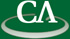## How much of mathematical invention can be automated?

### Bruno Buchberger, Johannes Kepler University, Linz

Friday May 3rd, 11:30am, IRMACS theatre, ASB 10900.

```Abstract: This talk proceeds on two floors. On the first floor, we give a short introduction
to the theory and method of Gröbner bases. This is a general algorithmic approach to the
solution of a fundamental problem in commutative algebra (polynomial ideal theory), which
prior to the solution in the speaker's PhD thesis in 1965, was open for several decades.
The Gröbner bases method is now available in all mathematical software systems like Mathematica
and Maple and has numerous applications in all areas of science and technology, in which
non-linear polynomial systems play a role. There will be practical examples how the method
can be used for problem solving in some of theses areas. The key insight on which the Gröbner
bases method hinges is the fact that, for handling the infinitely many "divergence" points
in the remaindering graph of polynomial systems, the check of finitely many critical
points in the reduction graph (the "S-polynomials") is sufficient.

On the second floor, we discuss the speaker's recent method of "lazy thinking" for the
automated invention and proof of mathematical theorems and algorithms. This method
systematizes and combines two simple heuristics: The use of formulae schemes (for proposing
potential theorems and algorithms) and the automated analysis of failures in proof attempts.

In the talk, we focus on the application of the lazy thinking approach to the
automated invention of algorithms. We will first illustrate the method in the standard example
of sorting: Starting from a specification of the sorting problem, using various algorithm
schemes, we arrive automatically at the various known sorting algorithms (in fact, at whole
classes of possible algorithms). We will then show that the method of lazy thinking is
powerful enough to synthesize automatically also algorithms for non-trivial problems, e.g.
the algorithm for the construction of Gröbner bases, notably the idea of "S-polynomials".
Thus, in a way, in this talk the speaker reports on how, in his mature age, he was able to
automate his mathematical inventive power of his young age.

The speaker will finally draw some conclusions on principal limitations for the automation
of mathematical invention; on the role of computers for mathematics and the role of mathematics
for computers; and the future and central role of mathematics and computer science for science,
technology, economy, and welfare in the 21st century. The talk addresses a general audience
of mathematicians and computer scientists. No special background in polynomial ideal theory
or automated reasoning is necessary.

```